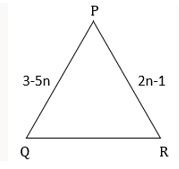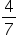Mathematics
Easy

Question

# Find the value of n if the given triangle is an equilateral triangle.## The correct answer is:### To find the value of n in the given equilateral triangle.The sides of an equilateral triangle are equal.3 - 5n = 2n - 17n = 4n =Therefore, using the property of equilateral triangle, the value of n is.

### Related Questions to study#### With Turito Foundation.#### Get an Expert Advice From Turito.